# Uncertainty ranking: SRC and SRRC¶

Standard Regression Coefficients (SRC) deal with analyzing the influence the random vector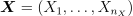has on a random variable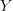which is being studied for uncertainty. Here we attempt to measure linear relationships that exist betweenand the different components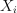.

The principle of the multiple linear regression model consists in attempting to find the function that links the variableto the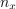variables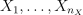by means of a linear model: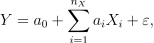wheredescribes a random variable with zero mean and standard deviation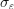independent of the input variables. If the random variablesare independent and with finite variance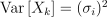, the variance ofcan be estimated as follows: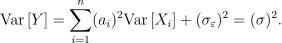From this we obtain the following coefficients: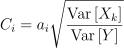The estimators for the regression coefficients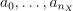, and the standard deviationare obtained from a sample of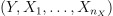. The SRC coefficients are defined as the estimators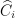of the coefficients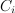: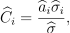where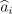denotes the estimate of the regression coefficient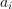,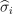denotes the empirical standard deviation of the sample of the input variableand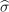denotes the empirical standard deviation of the sample of the output variable. The absolute value of this estimated contribution is by definition between 0 and 1. The closer it is to 1, the greater the impact the variablehas on the dispersion of.

The square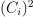, which is the contribution ofto the variance of, is sometimes described in the literature as the “importance factor”, because of the similarity between this approach to linear regression and the method of cumulative variance which uses the term importance factor.

It is a good idea to check the quality of the linear regression before estimating the SRC coefficients: if the linear regression model is a poor fit to the data, then the SRC coefficients are useless.

Note that if there exists a map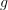such that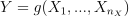, then the squared SRC coefficients are equal to Sobol’ indices.

Standard Rank Regression Coefficients (SRRC) are SRC coefficients computed on the ranked input variables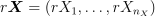and the ranked output variable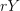. They are useful when the relationship betweenand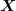is not linear (so SRC cannot be used), but only monotonic.